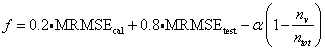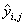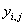Home News About Me Ph. D. Thesis Abstract Table of Contents 1. Introduction 2. Theory  Fundamentals of the Multivariate Data Analysis 2.1. Overview of the Multivariate Quantitative Data Analysis 2.2. Experimental Design 2.3. Data Preprocessing 2.4. Data Splitting and Validation 2.5. Calibration of Linear Relationships 2.6. Calibration of Nonlinear Relationships 2.7. Neural Networks  Universal Calibration Tools 2.8. Too Much Information Deteriorates Calibration 2.8.1. Overfitting, Underfitting and Model Complexity 2.8.2. Neural Networks and the Complexity Problem 2.8.3. Brute Force Variable Selection 2.8.4. Variable Selection by Stepwise Algorithms 2.8.5. Variable Selection by Genetic Algorithms 2.8.6. Variable Selection by Simulated Annealing 2.8.7. Variable Compression by Principal Component Analysis 2.8.8. Topology Optimization by Pruning Algorithms 2.8.9. Topology Optimization by Genetic Algorithms 2.8.10. Topology Optimization by Growing Neural Network Algorithms 2.9. Measures of Error and Validation 3. Theory  Quantification of the Refrigerants R22 and R134a: Part I 4. Experiments, Setups and Data Sets 5. Results  Kinetic Measurements 6. Results  Multivariate Calibrations 7. Results  Genetic Algorithm Framework 8. Results  Growing Neural Network Framework 9. Results  All Data Sets 10. Results  Various Aspects of the Frameworks and Measurements 11. Summary and Outlook 12. References 13. Acknowledgements Publications Research Tutorials Downloads and Links Contact Search Site Map Print this Page### 2.8.5.   Variable Selection by Genetic Algorithms

Genetic algorithms (GA) have become a popular optimization method as they often succeed in finding the best optimum in contrast to most common optimization algorithms. Genetic algorithms imitate the natural selection process in biological evolution with selection, mating reproduction and mutation. On the left-hand side of figure 5, the sequence of the different operations of a genetic algorithm is shown.

The parameters to be optimized are represented by a chromosome whereby each parameter is encoded in a binary string called gene. Thus, a chromosome consists of as many genes as parameters to be optimized. A population, which consists of a given number of chromosomes, is initially created by randomly assigning "1" and "0" to all genes. On the top right part of figure 5, the different terms are graphically shown for a population of 4 chromosomes with 4 genes (in the case of variable selection a gene contains only a single bit string for the presence and absence of a variable). The best chromosomes have the highest probability to survive evaluated by a so-called fitness function. The next generation is reproduced by selecting the best chromosomes, mating the chromosomes to produce an offspring population and by an occasional mutation. The evaluation and reproduction steps are repeated until a certain number of generations, until a defined fitness or until a convergence criterion of the population are reached. In the ideal case, all chromosomes of the last generation have the same genes representing the optimal solution. The theory and benefits of GA in variable selection have been described several times in literature - and will not be repeated here, as there are uncountable variants of the different genetic operators. Instead, a description of the GA implementation, which has been used in this work and its special features like the implementation of the fitness function and the other genetic operators will be further discussed and are illustrated on the right side of figure 5.figure 5:    Flow chart of a genetic algorithm (left side) and explanations of the different operations by the application of the genetic algorithm to a variable selection problem with 3 variables and neural networks for the evaluation of the fitness.

In this work, the initial population of the GA is randomly generated except of one chromosome, which was set to use all variables. The binary string of the chromosomes has the same size as variables to select from whereby the presence of a variable is coded as "1" and the absence of a variable as "0". Consequently, the binary string of a gene consists of only one single bit. After evolving the fitness of the population, the individuals are selected by means of the roulette wheel. Thereby, the chromosomes are allocated space on a roulette wheel proportional to their fitness and thus the fittest individuals are more likely selected. In the following mating step, offspring chromosomes are created by a crossover technique. A so-called one-point crossover technique is employed, which randomly selects a crossover point within the chromosome. Then two parent chromosomes are interchanged at this point to produce two new offspring. After that, the chromosomes are mutated with a probability of 0.005 per gene by randomly changing genes from "0" to "1" and vice versa. The mutation prevents the GA from converging too quickly in a small area of the search space.

A crucial point in using GA is the design of the fitness function, which determines what a GA should optimize. In the case of a variable selection for calibration, the goal is to find a small subset of variables, which are most significant for a regression. In this work, the calibration is based on neural networks for modeling the relationship between the input variables (many time-dependent sensor signals) and the responses (concentrations of the different analytes). Thus, the evaluation of the fitness starts with the encoding of the chromosomes into neural networks whereby "1" indicates that a specific variable is used and "0" that a variable is not used by the network. Then the networks are trained with a calibration data set and after that, a test data set is predicted. Finally, the fitness is calculated by a so-called fitness function f. In contrast to many GA for variable selection found in literature ,,-,, the fitness function used for the GA variable selections in this work takes into account not only the prediction error of test data but also partially the calibration error and primarily the number of variables used to build the corresponding neural nets:(16)

Thereby nv is the number of variables used by the neural networks, ntot is the total number of variables and MRMSE is the mean root mean square error of the calibration respectively test data. The MRMSE is defined in equation (17) with N as total number of samples predicted, M as total number of analytes,as predicted concentration of analyte j in sample i andas the corresponding known true concentration:(17)

The fitness function can be broken up into three parts. The first two parts correspond to the accuracy of the neural networks. Thereby MRMSEcal is based on the prediction of the calibration data used to build the neural nets, whereas MRMSEtest is based on the prediction of separate test data not used for training the neural networks. It was demonstrated in  that using the same data for the variable selection and for the model calibration introduces a bias. Thus, variables are selected based on data poorly representing the true relationship. On the other hand, it was also shown , that a variable selection based on a small data set is unlikely to find an optimal subset of variables. Therefore, a ratio of 1:4 between the influence of calibration and test data was chosen. Although being partly arbitrary this ratio should give as little influence to the calibration data as to bias the feature selection yet taking the samples of the larger calibration set partly into account. The third part of the fitness function rewards small networks using only few variables by an amount proportional to the parameter a. The choice of a influences the number of variables used by the evolved neural nets. A high value of a results in only few variables selected for each GA whereas a small value of a results in more variables being selected.

As the initial weights of a neural network are randomly set, the network finds another local minimum of the error surface for each calibration run with a slightly different performance of prediction. In order to reduce the variance of the error of prediction due to random weight initialization the fitness is averaged in expression (17) over 20 training and prediction sessions per network topology (evaluation of 20 parallel neural networks with different initial weights).

 Page 52 © Frank Dieterle, 03.03.2019